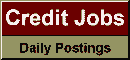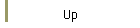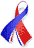DefaultRisk.com the web's biggest credit risk modeling resource.doi> search: A or B Export citation to:- HTML- Text (plain)- BibTeX- RIS- ReDIF

Negative Probabilities in Financial Modeling

by Mark Burgin of the University of California, Los Angeles, and
Gunter Meissner of the University of Hawaii

January 2010

Abstract: We first define and derive general properties of negative probabilities. We then show how negative probabilities can be applied to modeling financial options such as Caps, Floors and Swapoptions. In trading practice, these options are typically valued in a Black-Scholes-Merton framework assuming a log-normal distribution for the underlying interest rate. However, in some cases, such as the 2008/2009 financial crisis, interest rates can get negative. Then the log-normal distribution is inapplicable. We show how negative probabilities can be applied to value interest rate options in a log-normal framework implying a positive probability for negative interest rates. A model in VBA, which prices Caps, Floors and Swapoptions with negative probabilities, is available upon request.

Keywords: Negative probabilities, negative interest rates, Caps, Floors, Swaptions

Books Referenced in this paper:  (what is this?)